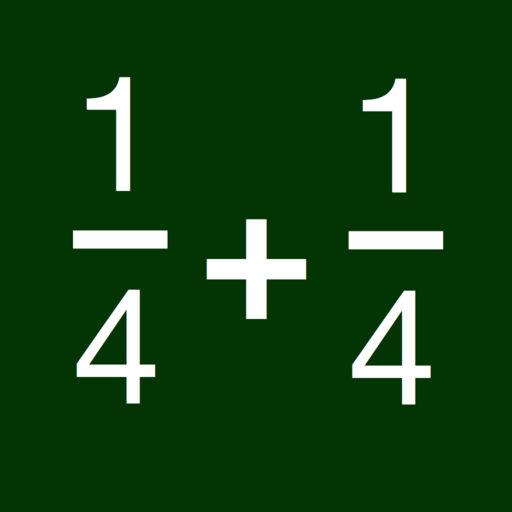Fraction Drills# Fraction Drills

## William Jockusch

\$1.99
0

0 Ratings

Update Date

2017-03-22

Size

4.0 MB

Fraction Drills Description
Having problems learning to work with fractions? Or just want a refresher? This app will help you do that!

The available problem types are organized into "levels", so you can start with easier problems, and work your way up to harder ones. There is a "test" mode that lets you test yourself to see how much you have learned.

Available problem types:

level 1:
* simplify (e.g. 4/6=2/3)
* change denominator (e.g. rewrite 2/3 as 4/6).
* convert fraction to mixed number (e.g. 3/2 is the same as 1 1/2)
* convert mixed number to fraction (rewrite 1 1/2 as 3/2).

Level 2:
* add and subtract (same denominator, e.g. 1/6+2/6)
* multiply and divide by whole numbers (e.g. 2/5*2)
* Invert, and answer with a fraction (e.g. 1 / (2/5) = 5/2 )

Level 3
* Multiply and divide fractions
* Invert, and answer with a mixed number (e.g. 1/ (2/5) = 2 1/2)
* Find the least common denominator (e.g., given 1/4 and 5/6, LCD is 12)

Level 4
* Add mixed numbers (e.g. 1 1/2 + 2 1/2)
* Subtract mixed numbers
* Multiply mixed numbers
* Divide mixed numbers
Price:
\$1.99
Version:
2.0
Size:
4.0 MB
Genre:
Education
Update Date:
2017-03-22
Developer:
William Jockusch
Language:
English
More